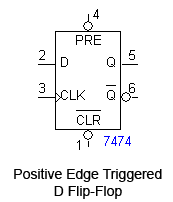# Flip-Flops and Latches

Flip-flops are a useful type of digital device that can store binary states, or be used as a sort of digital toggle switch. Flip-flops can be built up from distinct logic gates, but they can easily be bought in packaged chips. The flip-flop is a digital device, so its output (labeled with a Q in schematic diagrams) will take on the value of either 1 (high) or 0 (low). Many flip-flops will also provide the complementary output (an inverted output).

## Edge Triggering

Because the state of a flip-flop often depends on the previous state of a circuit (for example, the output of one flip flop may be the input to another), and because each flip-flop and logic gate needs a certain amount of time to switch its output, we usually clock the devices, that is, we synchonize all the flip-flops to change states at the same time with a clocked pulse. Flip-flops are edge triggered; they either change states when the clock goes from 0 to 1 (positive/rising edge) or when the clock goes from 1 to 0 (negative/falling edge).

The symbols used for positive and negative edge triggering on flip-flops:

## Asynchonous Inputs

Many flip-flops will also have a clear (CLR) and preset (PRE) terminal. These inputs are typically inverted, so they are active when the input signal is low (Active Low Input). The CLR and PRE signals can be asserted any time and don't have to be edge triggered; they will override any other inputs, including the clock.

The symbols used for clear and preset (the bubble indicates an inverted signal):

Truth Table for CLR and PRE (active low)
PRE CLR Output
0 0 Error state
0 1 1
1 0 0
1 1 Depends on inputs and clock

## Types of flip-flops

There are several types of flip-flops but the two most important kind are the D and J-K flip-flops.

### D flip-flop

Symbol for the D flip-flop:The D (Data) flip-flop has an input D, and the output Q will take on the value of D at every triggering edge of the clock pulse and hold it until the next triggering pulse. The D flip-flop is usually positive edge triggered.

The truth table for a positive edge triggered D flip-flop:

D CK Q
0 0
1 1
X 0,1 Q0

(↑ indicates a rising edge on the clock pulse; X indicates that it has no effect on outcome)

Timing diagram for the positive edge triggered D flip-flop:

### JK flip-flop

Symbol for the JK flip-flop:The JK flip-flop has two inputs, labeled J and K. J corresponds to a "set" signal, and K corresponds to a "reset" signal.

At the triggering edge:

If J is 1 and K is 0, Q is 1.

If J is 0 and K is 1, Q is 0.

If J and K are both 0, the output stays the same as it was before.

If J and K are both 1, the output is inverted.

The JK flip-flop is usually negative edge triggered.

The truth table for a negatively triggered JK flip-flop:

J K CK Q
0 0 Q0
0 1 0
1 0 1
1 1 Q'0
X X 0,1 Q0

The timing diagram for the negatively triggered JK flip-flop:

## Latches

Latches are similar to flip-flops, but instead of being edge triggered, they are level triggered.

The most common type of latch is the D latch. While CK is high, Q will take whatever value D is at. When CK is low, Q will latch onto the last value it had before CK went low, and hold it until CK goes high again.

Timing diagram for the D latch: